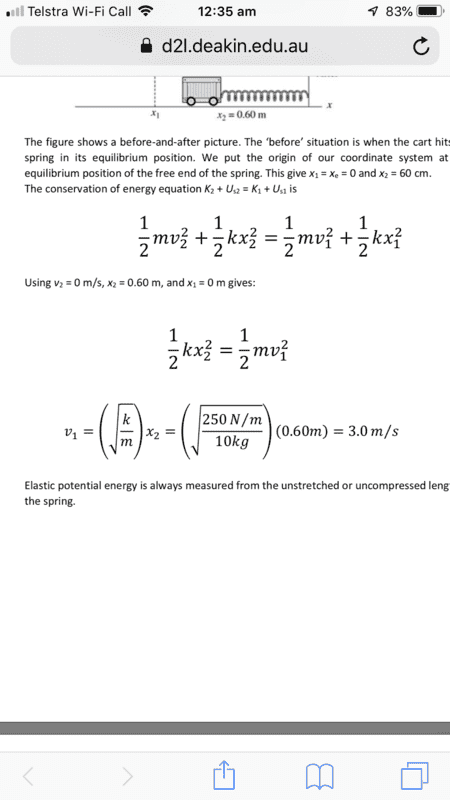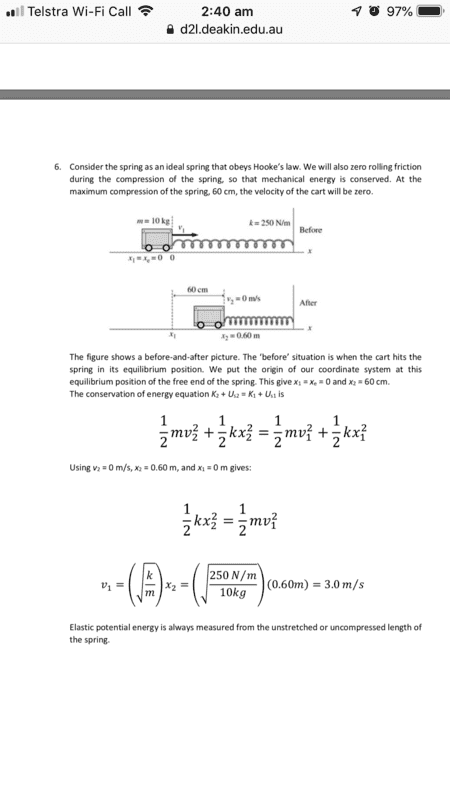# Conservation of energy: Question about simplifying this equation (cart and spring)

Homework Statement:
Unable to solve for solution.
Relevant Equations:
Kinematic energy and spring energy
Am unable to understand how in the final answer the X2^2 gets canceled out without being inside the radical. It doesn’t make sense to me.

Any help explaining would be much appreciated as I am stuck.Last edited by a moderator:Full visibility

Homework Helper
Gold Member
Can you solve the equation$$\frac{1}{2}kx_2^2=\frac{1}{2}mv_1^2$$for ##v_1## in terms of the other quantities? What do you get?

Can you solve the equation$$\frac{1}{2}kx_2^2=\frac{1}{2}mv_1^2$$for ##v_1## in terms of the other quantities? What do you get?

Yes it’s just x2 to take out the 1/2 after factoring on both sides then divide by M.

I’m more trying to understand why the x is left outside and isn’t inside the radical also. In this one here it’s left outside when usually it’s left inside.

Is there a particular reason?

Homework Helper
Gold Member
The only reason I can think of is "simplify as much as you can to enhance readability and ease of calculation". Remember that $$\omega=\sqrt{\frac{k}{m}}$$ so that$$v_1=\sqrt{\frac{k}{m}}x_2=\omega \ x_2$$ The last result is simpler and more readable. Besides, if you want to find a number for ##v_1## and you put ##x_2^2## under the radical, you will have to square ##x_2## and then take its square root.

Homework Helper
The only reason I can think of is "simplify as much as you can to enhance readability and ease of calculation". Remember that $$\omega=\sqrt{\frac{k}{m}}$$ so that$$v_1=\sqrt{\frac{k}{m}}x_2=\omega \ x_2$$ The last result is simpler and more readable. Besides, if you want to find a number for ##v_1## and you put ##x_2^2## under the radical, you will have to square ##x_2## and then take its square root.
Note that ##v^2=x^2##, simplifies to ##v=\pm x##. Be careful not to simplify one of the two solutions out of existence.

•